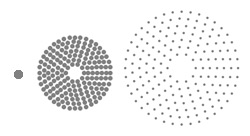s Physicist Uses Mathematica to Develop an Optics Program at CERN
Customer Stories ›

# Physicist Uses Mathematica to Develop an Optics Program at CERN

"Its programming language is the distinctive feature of Mathematica. List processing, functional programming, and object orientation are of general interest for any applications, symbolic or not. In accelerator controls, the symbolic aspect is limited, but the linkage of algorithms to databases and the C programs of graphic interfaces is both efficient and elegant using Mathematica and MathLink."
The Mathematica Edge
• Symbolic—solving equations and discovering general structures
• Graphic—solution visualization
• Programming—efficient list and matrix manipulation

Geneva, Switzerland—Under the city, 100 meters deep, lies a particle accelerator with a 27-kilometer circumference used to study subatomic particles. By creating collisions at fantastic energies, it is possible to create particles that otherwise occurred only during the violence of the Big Bang.

A successful experiment requires precise control and focusing of relativistic beams. Bruno Autin, a physicist at CERN, used Mathematica to create an optics program to accomplish this delicate, yet complex task.

"Optics calculations involve heavy matrix manipulations and the solving of high-degree algebraic equations. The symbolic algebra capabilities of Mathematica are an ideal tool to calculate optical modules," Autin said.